# Can i please get help with problem 3? please, if possible, answer in a text format....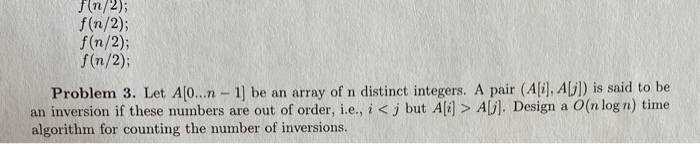JN/2) f(n/2); f(n/2); f(n/2); Problem 3. Let A[O...n-1) be an array of n distinct integers. A pair (Ali), A[]) is said to be an inversion if these numbers are out of order, i.e., i<j but A[i] > AG). Design a O(n log n) time algorithm for counting the number of inversions.

#include<bits/stdc++.h>
using namespace std;
int merge(int a[],int st,int mid,int end)
{
int inv=0;
int temp[end-st+1];
int l=0;
int i=st;
int j=mid+1;
while(i<=mid && j<=end)
{
if(a[i]<a[j])
{
temp[l++]=a[i++];
}
else
{
temp[l++]=a[j++];
inv+=(mid-i+1);
}
}
while(i<=mid)
temp[l++]=a[i++];
while(j<=end)
temp[l++]=a[j++];
for(i=0;i<l;i++)
a[st+i]=temp[i];
return inv;
}
int countInversions(int a[],int st,int end)
{
int inv=0;
if(st<end)
{
int mid=(st+end)/2;
inv=countInversions(a,st,mid);
inv+=countInversions(a,mid+1,end);
inv+=merge(a,st,mid,end);
}
return inv;
}
int main()
{
int n,i;
cin>>n;
int a[n];
for(i=0;i<n;i++)
cin>>a[i];
cout<<countInversions(a,0,n-1);
}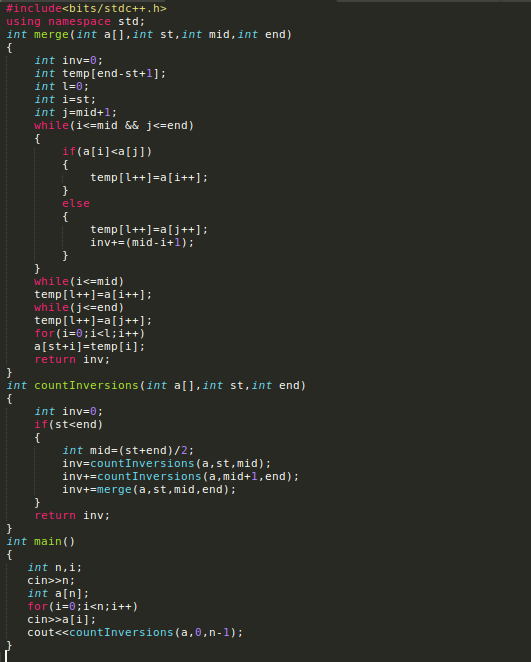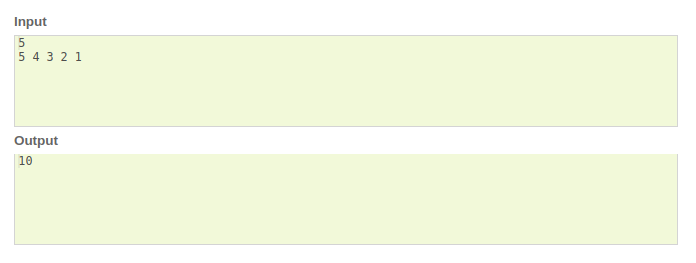Time Complexity:- O(nlogn)

Please upvote if you found this solution useful and comment for any doubts.

#### Earn Coin

Coins can be redeemed for fabulous gifts.

Similar Homework Help Questions
• ### ALgorithm problem

Recall the problem of ¯nding the number of inversions. As in the text, we are given asequence of numbers a1; : : : ; an, which we assume are all distinct, and we de¯ne an inversionto be a pair i < j such that ai > aj .We motivated the problem of counting inversions as a good measure of how di®erent twoorderings are. However, one might feel that this measure is two sensitive. Let's call a pair asigni¯cant inversion if...

• ### ALGORITHM PROBLEM: A) Significant Inversions: We are given a sequence of n arbitrary but distinct real...

ALGORITHM PROBLEM: A) Significant Inversions: We are given a sequence of n arbitrary but distinct real numbers <a1 , a2 ,..., an>. We define a significant inversion to be a pair i < j such that ai > 2 aj . Design and analyze an O(n log n) time algorithm to count the number of significant inversions in the given sequence. [Hint: Use divide-&-conquer. Do the “combine” step carefully] B) The Maximum-Sum Monotone Sub-Array Problem: Input: An array A[1..n] of...

• ### the question from the course COMP 4040 that Analysis of Algorithms if you want to answer it by code please use C or C++...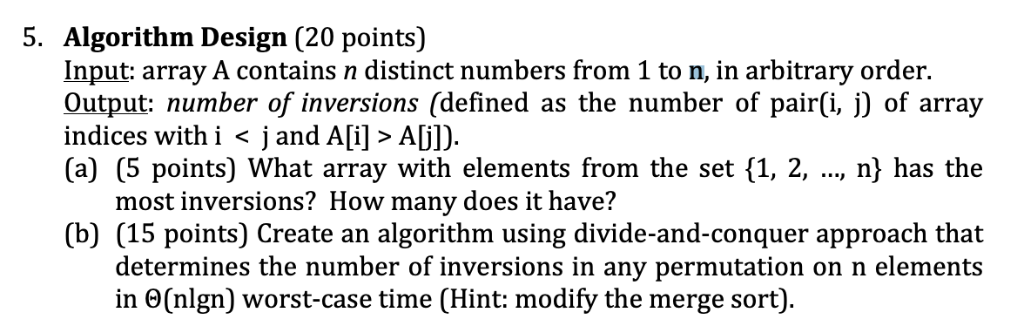the question from the course COMP 4040 that Analysis of Algorithms if you want to answer it by code please use C or C++ 5. Algorithm Design (20 points) Input: array A contains n distinct numbers from 1 to n, in arbitrary order. Output: number of inversions (defined as the number of pair(i, j) of array indices with i < j and A[i] > Aj]) (a) (5 points) What array with elements from the set {1, 2, ..., n) has...

• ### Divide and Conquer

Recall the problem of finding the number of inversions. As in the test, we are given a sequence of n numbers a1, …, an, which we assume are all distinct, and we define an inversion to be a pair of i < j such that a1 >aj.We motivated the problem of counting inversion as a good measure of how difference two orderings are. However, one might feel that this measure is too sensitive. Let’s call a pair a significant inversion if i...

• ### Design and analysis of algorithms Type in answer Problem 5. Given a sorted array of distinct...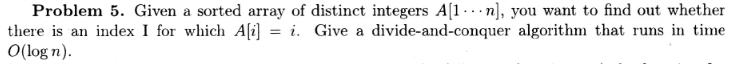Design and analysis of algorithms Type in answer Problem 5. Given a sorted array of distinct integers A[1- -n], you want to find out whether there is an index I for which Ai-i. Give a divide-and-conquer algorithm that runs in time O(log n)

• ### Collaborative ﬁltering is a technique for generating recommendations, such as suggestions for products, songs, movies, news...

Collaborative ﬁltering is a technique for generating recommendations, such as suggestions for products, songs, movies, news stories, and so on. The idea is to identify other users who have similar preferences, and recommend to you things that have been popular with them. It requires a formal notion of “similarity” between users, and some of the essence of this is captured by the problem of counting the number of inversions in an array. An inversion in an array A[1,...,n] is a...

• ### 4 Problem 4 -Extra Credit Given an array A, we say that elements A and Al...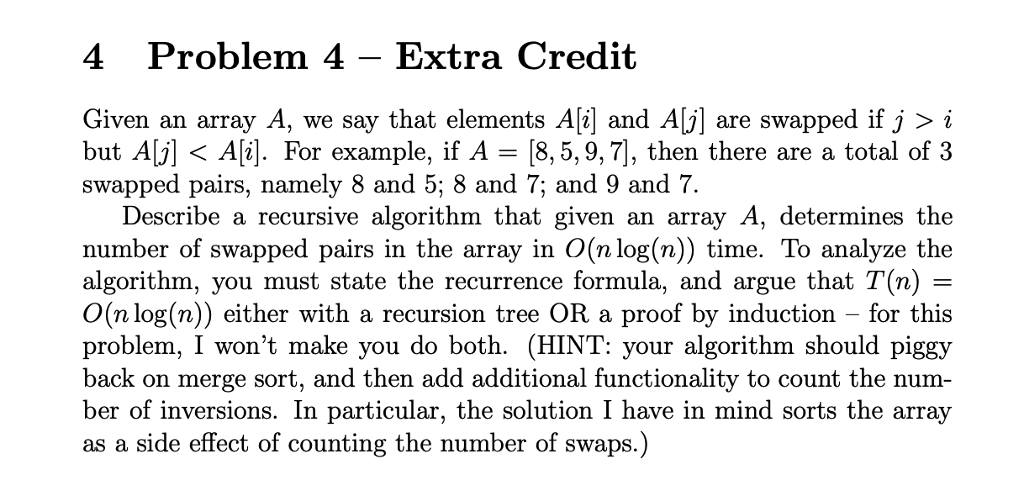4 Problem 4 -Extra Credit Given an array A, we say that elements A and Al are swapped if J >「 but Alj]< A[i]. For example, if A - [8,5,9,7], then there are a total of3 swapped pairs, namely 8 and 5; 8 and 7; and 9 and 7 Describe a recursive algorithm that given an array A, determines the number of swapped pairs in the array in O(n log(n)) time. To analyze the algorithm, you must state the recurrence...

• ### 6. Consider the following basic problem. You're given an array A consisting of n integers A,...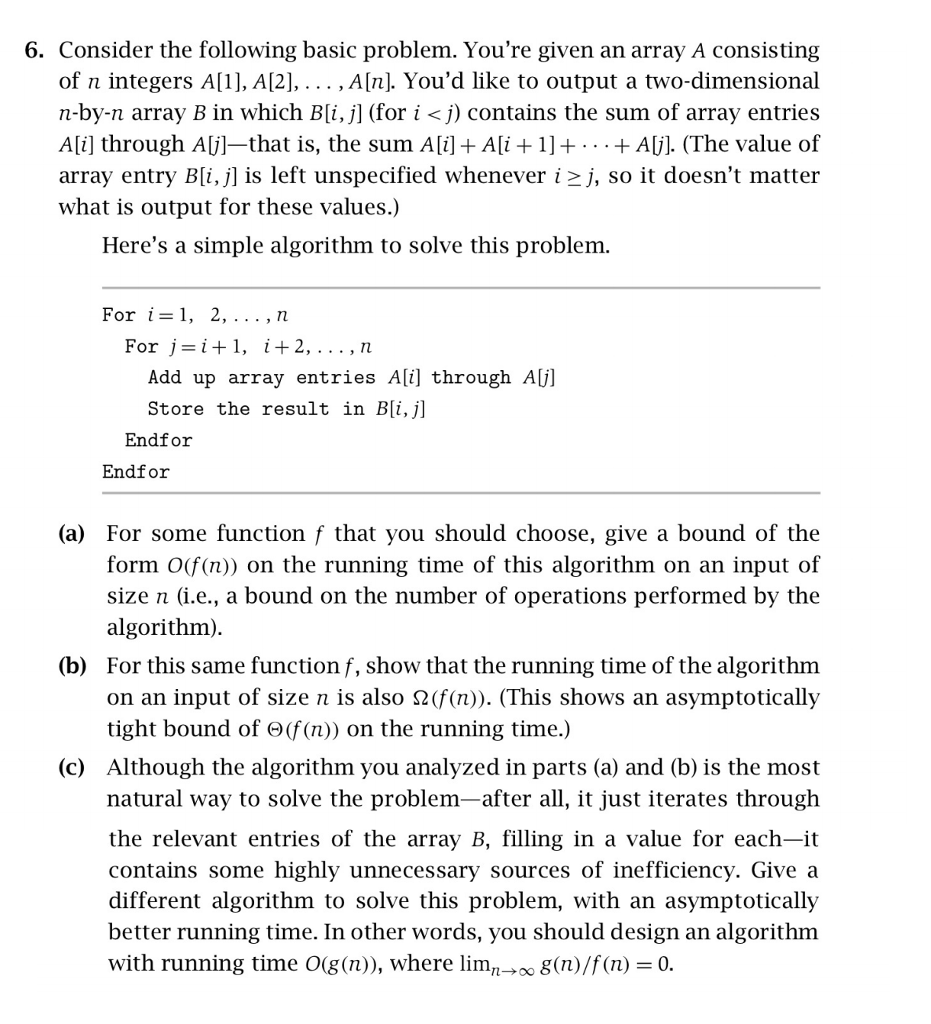6. Consider the following basic problem. You're given an array A consisting of n integers A, A, , Aln]. You'd like to output a two-dimensional n-by-n array B in which B[i, j] (for i <j) contains the sum of array entries Ali] through Aj]-that is, the sum A[i] Ai 1]+ .. +Alj]. (The value of array entry B[i. Λ is left unspecified whenever i >j, so it doesn't matter what is output for these values.) Here's a simple algorithm to...

• ### Can someone please help me in solving question in attached image. Thanks Suppose that an array...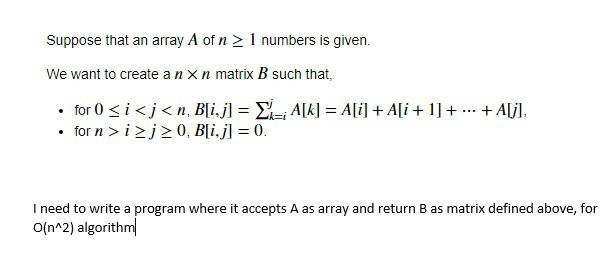Can someone please help me in solving question in attached image. Thanks Suppose that an array A of n 1 numbers is given. We want to create a n x n matrix B such that, for 0 i<j<n, Bli.j] AIk] = Ali]Ali+1] for n ij 0, B[i.j] 0 +Aljl k-i I need to write a program where it accepts A as array and return B as matrix defined above, for O(n 2) algorithm

• ### maybe use induction to prove? Problem 2: Let p-p.Pn be a permutation considered in its one-line notation. An inversion in p is a pair 1 i<jS n such that j appears to the left of i in p (i.e.,...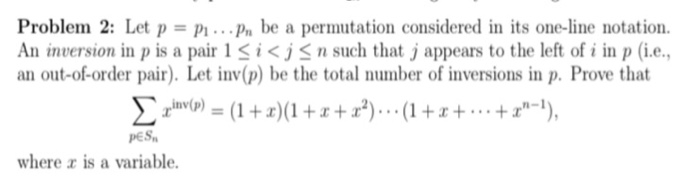maybe use induction to prove? Problem 2: Let p-p.Pn be a permutation considered in its one-line notation. An inversion in p is a pair 1 i<jS n such that j appears to the left of i in p (i.e., an out-of-order pair). Let inv(p) be the total number of inversions in p. Prove that PES where z is a variable. Problem 2: Let p-p.Pn be a permutation considered in its one-line notation. An inversion in p is a pair 1...